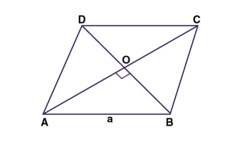# Adjacent sides of a parallelogram are equal and one of diagonals is equal to any one of the sides of this parallelogram. Show that its diagonals are in the ratio square root of 3 :1.Here ABCD is a parallelogram.

The Opposite sides of the parallelogram ABCD are equal.

GIven parameters

AB = DC and AB = BC

Now AB = BC = CD = DA

Also, AB = BC = CD = DA = AC (Diagonal is equal to the side)

ABCD is a Rhombus.

In a Rhombus the diagonals bisect each other at 90 degrees, so we can say that

AB = BC = CD = DA = AC = a

Consider ΔOAB,

According to Pythagoras theorem,

AB² = OA² + OB²

a² = OB² + (a/2)²

OB² = a² – a²/4

OB = (a√3)/2

∴ BD = 2. OB

⇒ BD = a√3

The length of diagonals are a and a√3.

The ratio of the Diagonals are

BD/AC = a√3/a

BD/AC = a/1

∴ BD : AC = √3 : 1(9)(6)